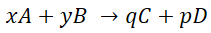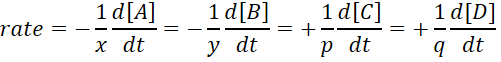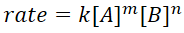# 16.1 Rate expression and reaction mechanism

### Rate equation (Rate law)

• Consider reaction:
•• Where x, y, p, q are stoichiometry coefficients
• The rate equation is:
•• In general the rate law is expressed as such:
•• Where m and n are the orders with respect to their reactants
• Order of reaction = m + n
• DO NOT USE STOICHIOMETRY COEFFICIENTS AS “m” AND “n” IN THIS EQUATION
• Rate equations can only be determined experimentally because orders can only be deduced empirically

### Molecularity and rate-determining step (slow-step) of a reaction

• Reaction mechanism: The sequence of steps outlining the reaction pathway from reactants to products
• Elementary steps: Any individual step in the reaction mechanism
• Molecularity: the number of molecules or atoms involved as reactants in the elementary reaction
• Unimolecular: Single molecule or atoms involved in an elementary step
• Bimolecular: Two molecules or atoms involved in an elementary step
• Each elementary step has its own rate constant, k, and its own activation energy Ea
• The rate of reaction depends on the slow step or the rate-determining step (RDS)
• Catalysts alter reaction mechanism by introducing a step with a lower activation energy

### Deduction of a rate equation

1. Decide on which step is the RDS
2. Deduce rate equation for the RDS

### Graphical representations of reactions